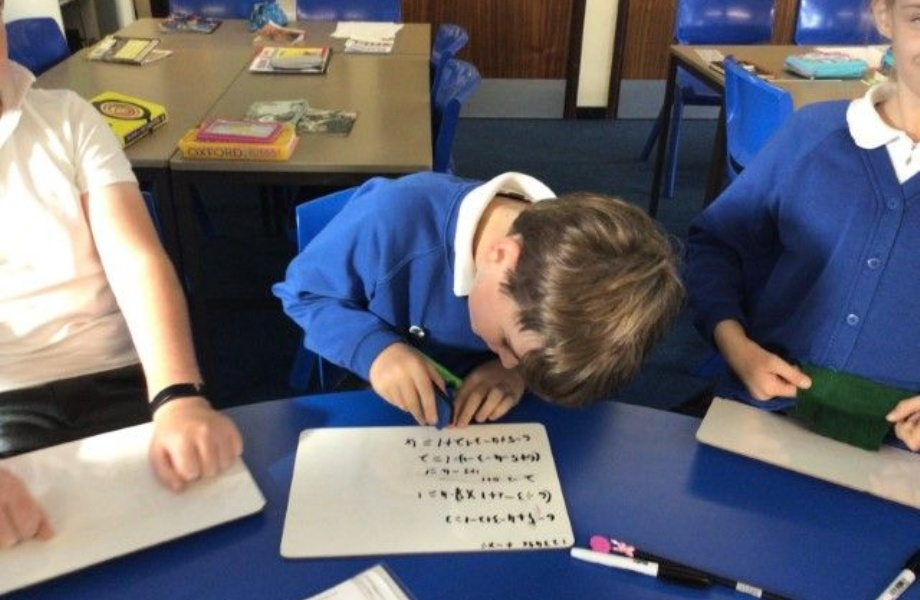# Stanton St QuintinPrimary School

Discovering Together

# Y6 have been learning about BIDMASYear 6 have learnt that the order of operations in an equation matter.

BIDMAS helps you to remember the order that you need to do your mathematical calculations where there is more than one mathematical operator (any combination of brackets, indices, division, multiplication, addition, subtraction).

This easily remembered acronym stands for:

B rackets
I ndices (powers of)
D ivision
M ultiplication
A ddition
S ubtraction

Well done Year 6 there were some tricky calculations!